# 通过流体动力学研究煎饼制作的最佳方法

2019年 11月 6日

### 煎饼制作和最佳覆盖问题

• 搅拌器
• 冰箱
• 涂抹器
• 锅铲（如果需要，用于翻转）
• 不粘锅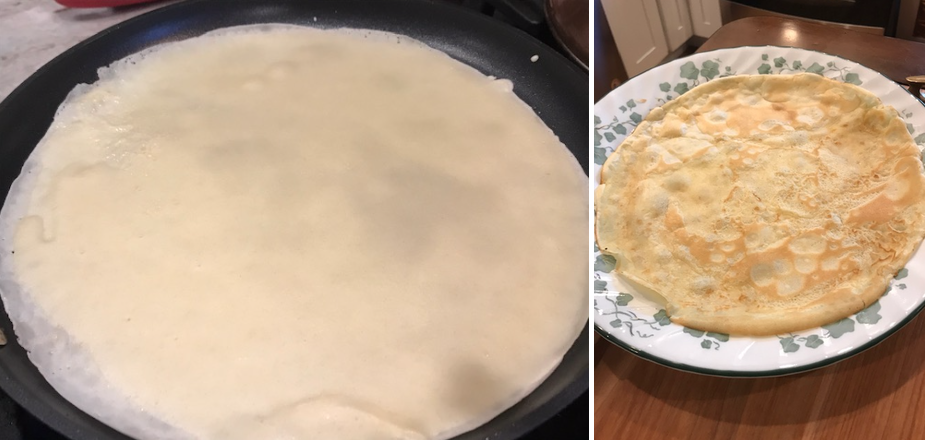### 使用COMSOL Multiphysics®在面盘上模拟面糊的流动和硬化

Boujo说：“从一个侧面项目开始，很快就会发现它与其他有关均匀厚度液体薄膜的都有关系。” 他解释说，这对于许多需要具有非常薄且均匀涂层的工业过程至关重要，例如智能手机显示屏，太阳能电池，电子电路板等。

\theta(t) = A_1\sin(2\pi t/T_1), \quad \beta(t)=A_2\sin(2\pi t/T_2),

{U}
(t_f) = \int\int \left(h(x,y,t_f)-h_\mathrm
{opt}\right)^2dxdy,

{ J}_t ={\cal U}(t_f) + \gamma {\cal C}, \quad \mathrm{} \quad { C} = \frac{1}{t_f}\int\left(\theta(t)^2+\beta(t)^2\right)dt .

### 评估流体动力学模拟的结果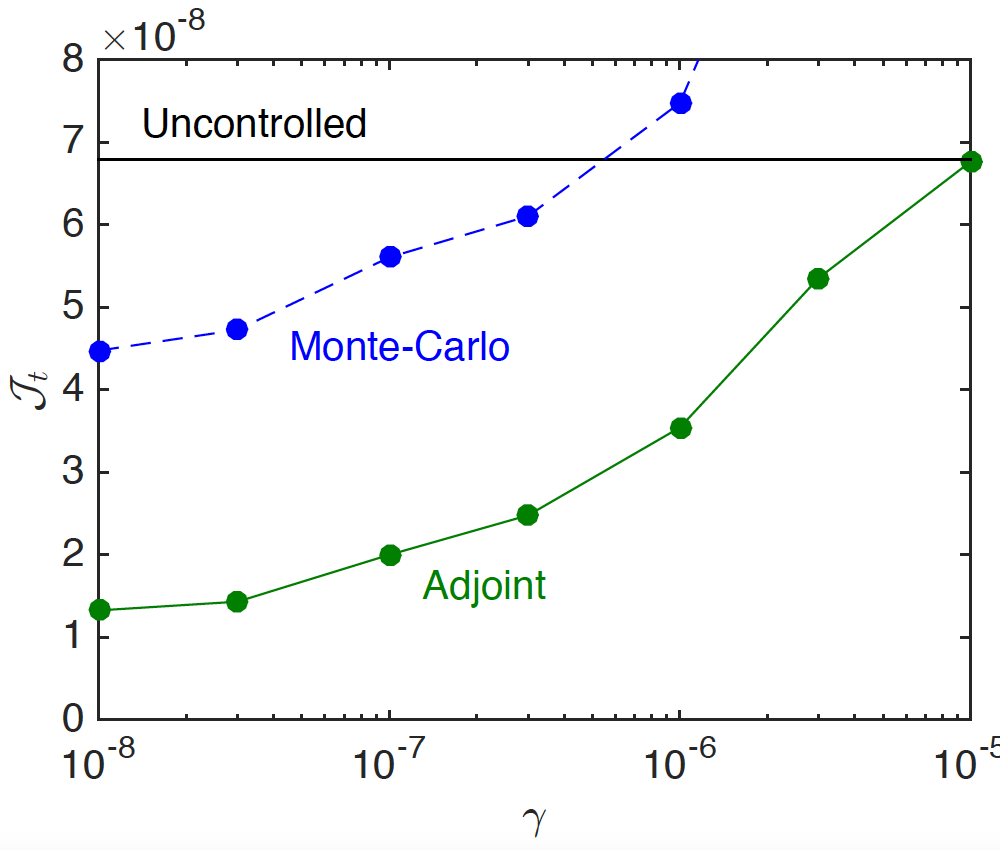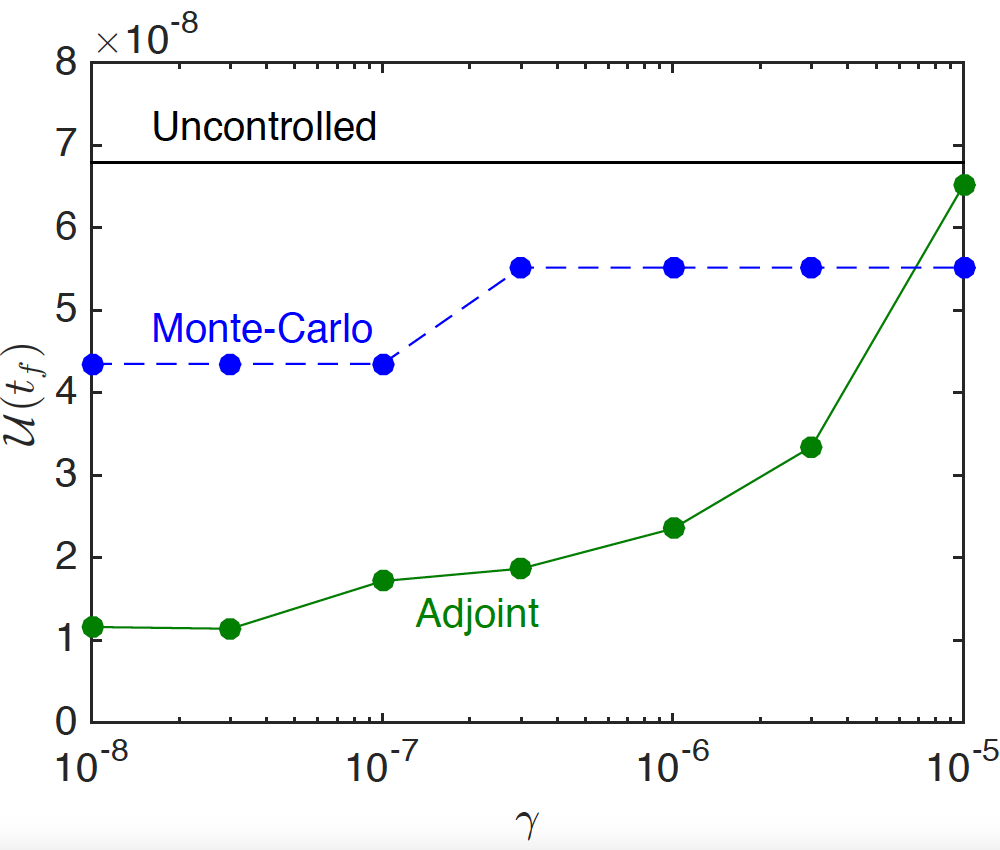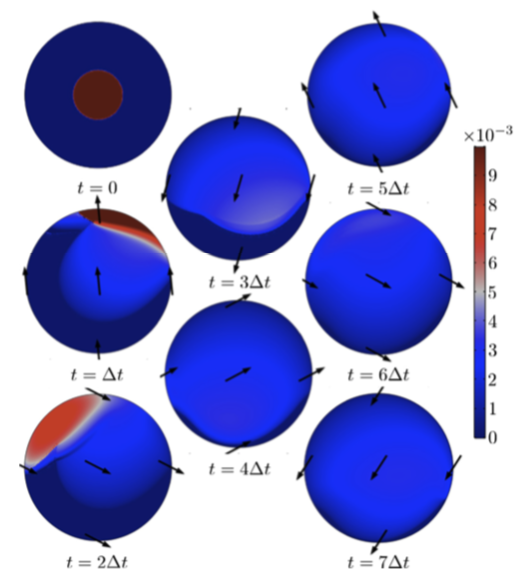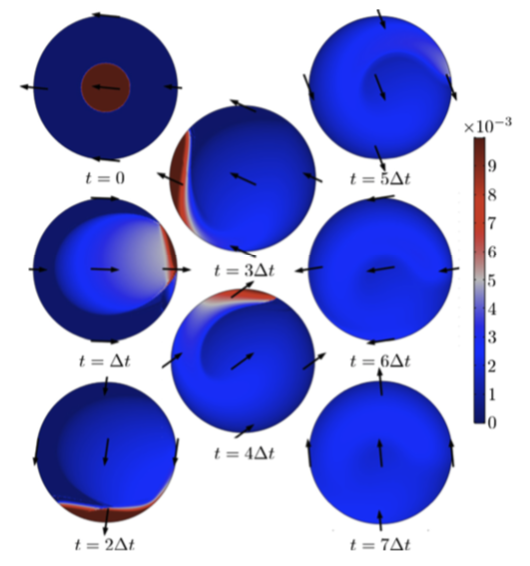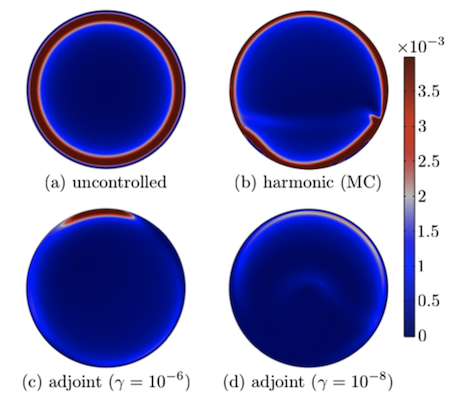### 煎饼的最佳制作方法是什么？都在手腕上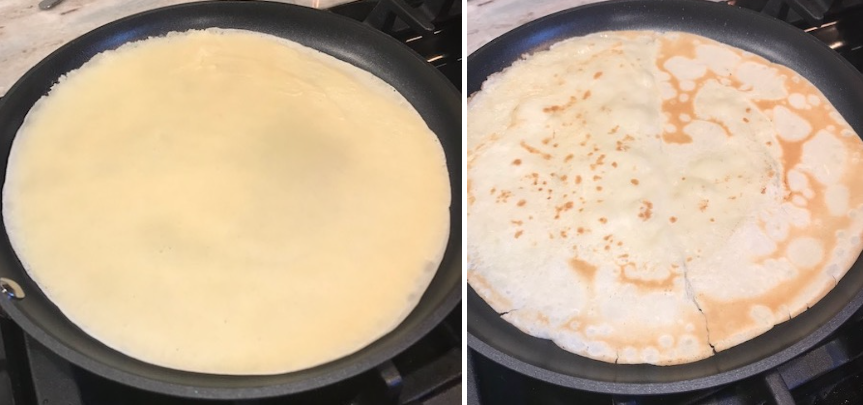### 进一步的思考

Boujo 和 Sellier 意识到他们从研究结果中获得的最佳平底锅晃动动作与厨师已经做过的动作非常相似。他们说：“至少在面皮的制作过程中，能让人们直观地感觉煎饼就是这样制作的，这已经很好了。”

### 下一步

MATLAB是The MathWorks，Inc.的注册商标。